# RD Sharma Solutions For Class 7 Maths Chapter - 1 Integers

RD Sharma Solutions For Class 7 Chapter 1 Integers PDF are provided here. Students can download the pdf of these solutions from the given links. Class 7 is a stage where several important topics are introduced. These crucial topics are discussed here in a particular way. The solutions are provided in accordance with the latest syllabus of CBSE which, in turn, help the students to build a strong foundation and secure excellent marks in their board exams. Therefore, we at BYJU’S provide answers to all questions uniquely and briefly. The solutions to all questions in RD Sharma books are given here in a step by step way to help the students understand effectively. In this chapter, we will learn about multiplication and division of integers and various properties of these operations on integers.

## Download the PDF of RD Sharma For Class 7 Maths Chapter 1 Integers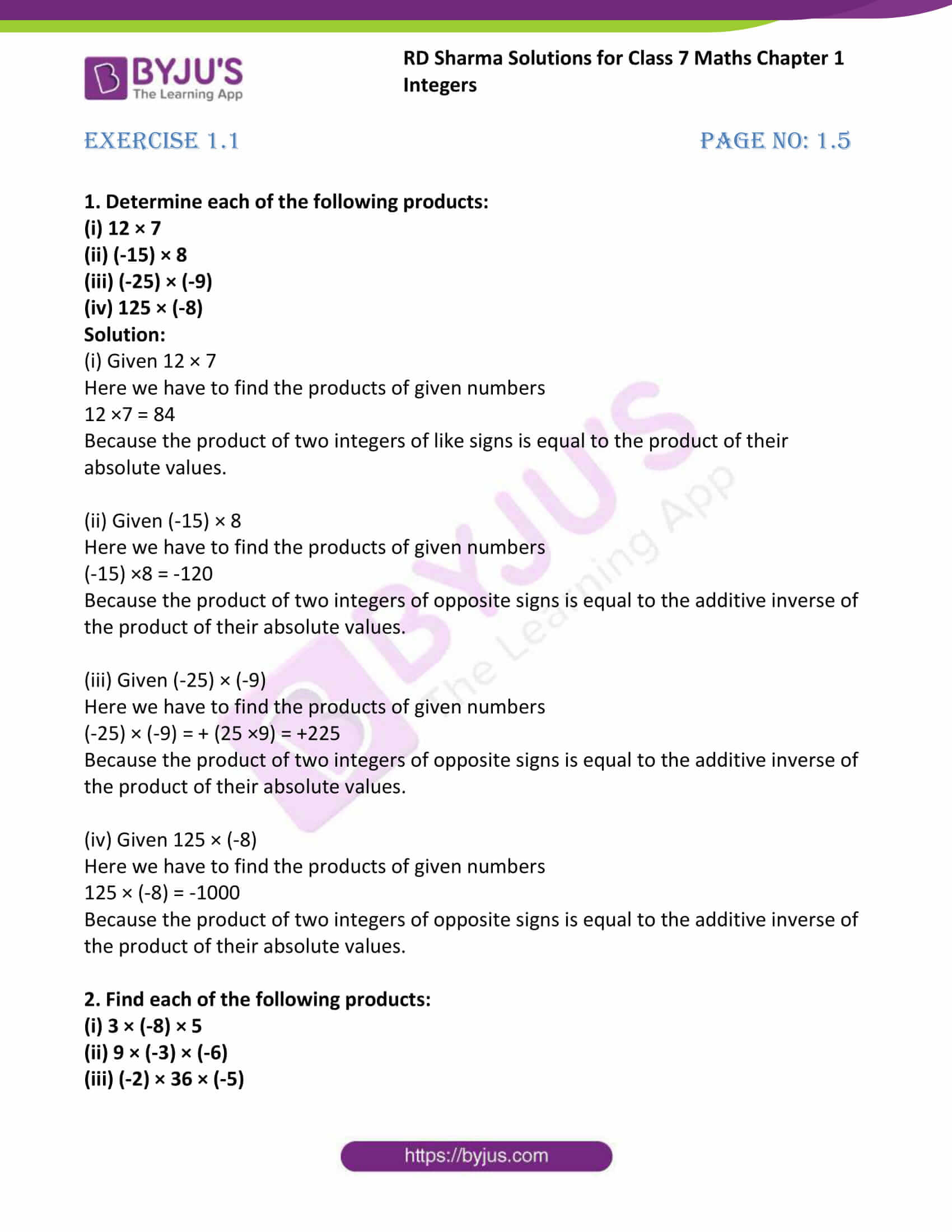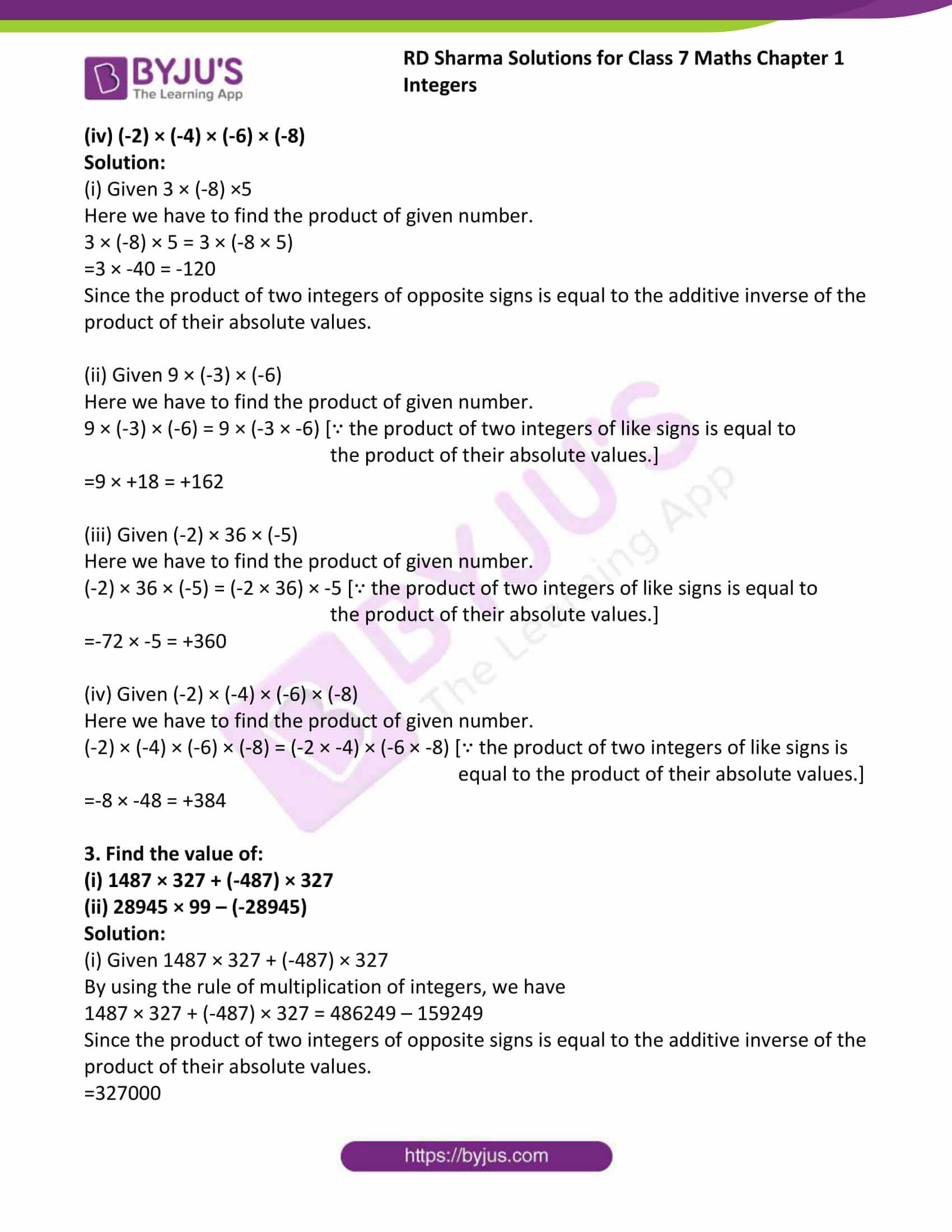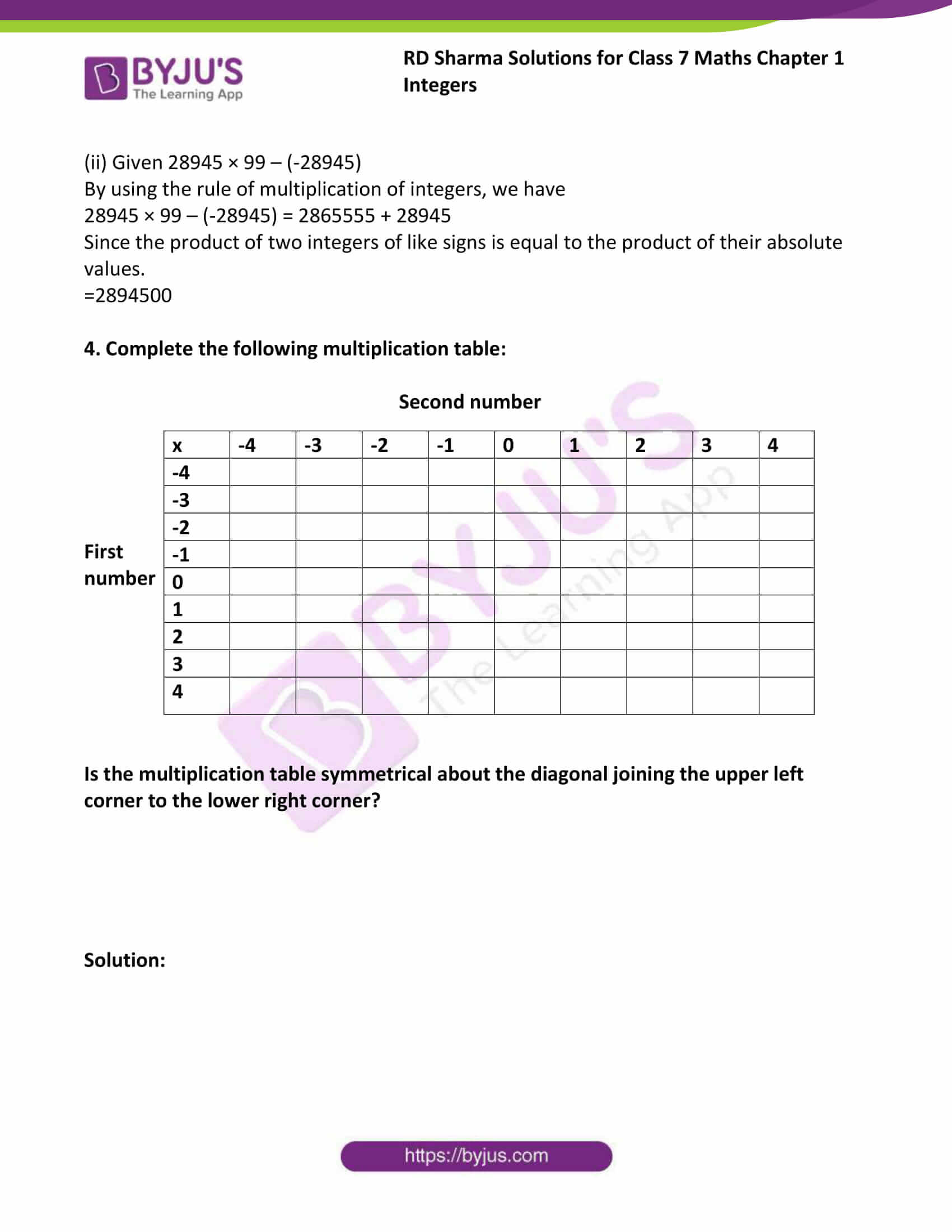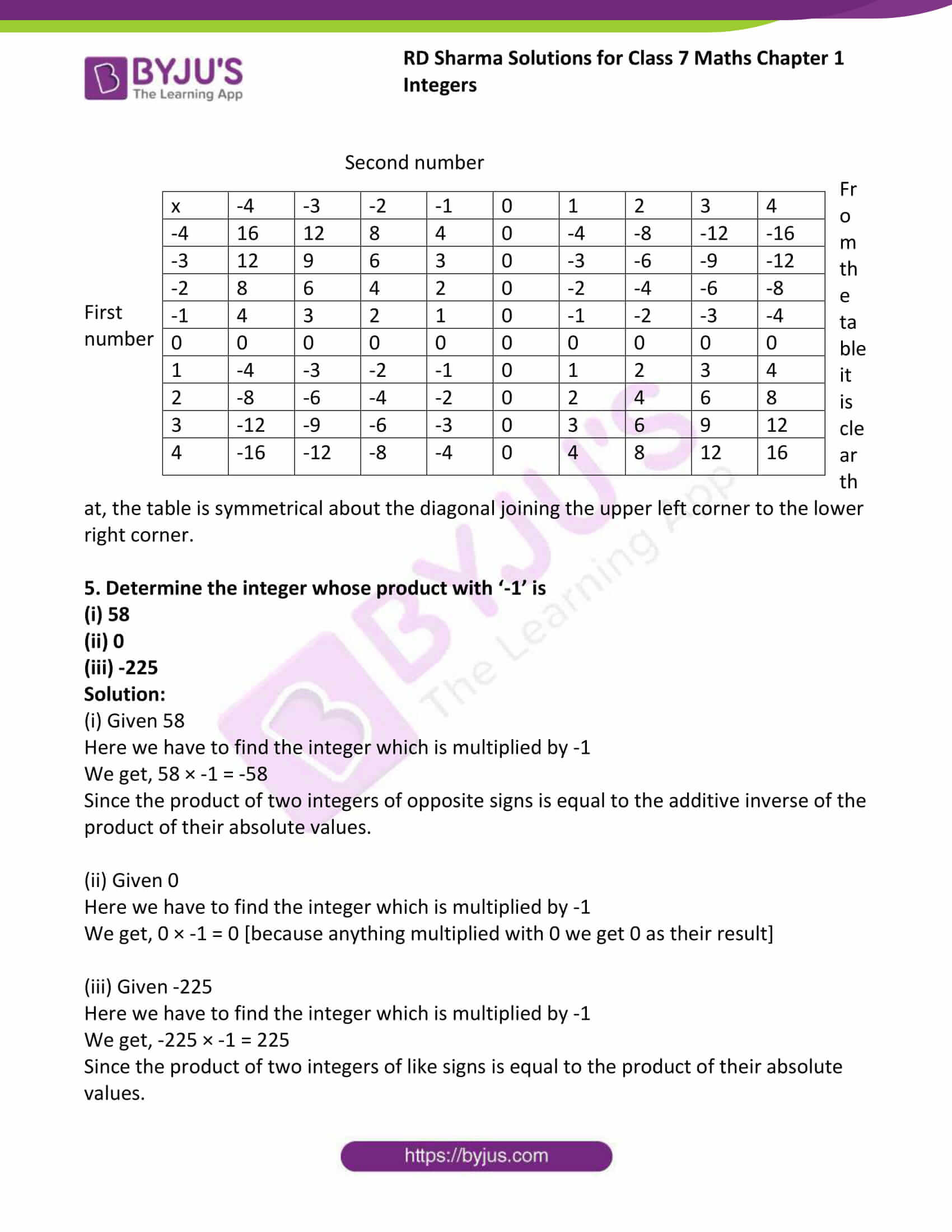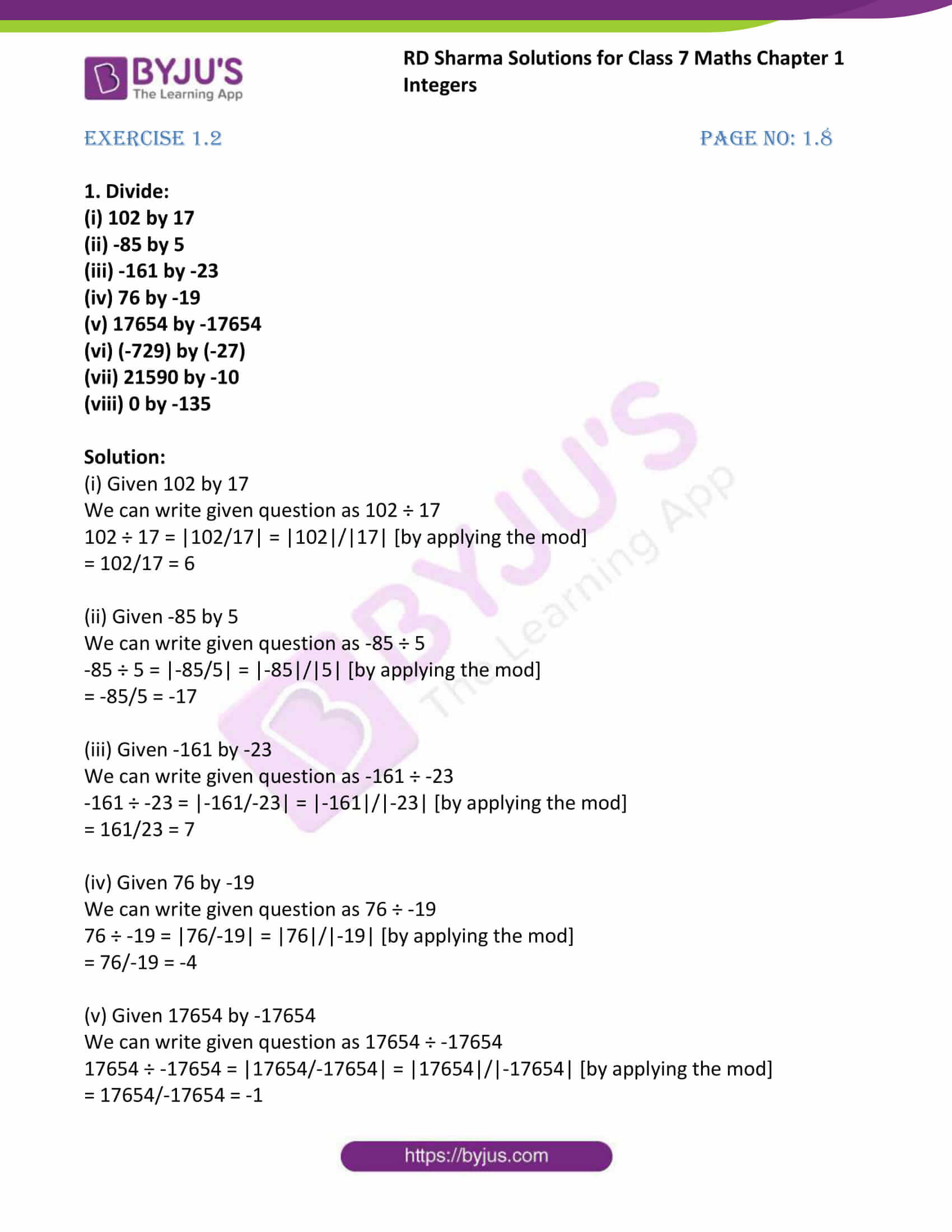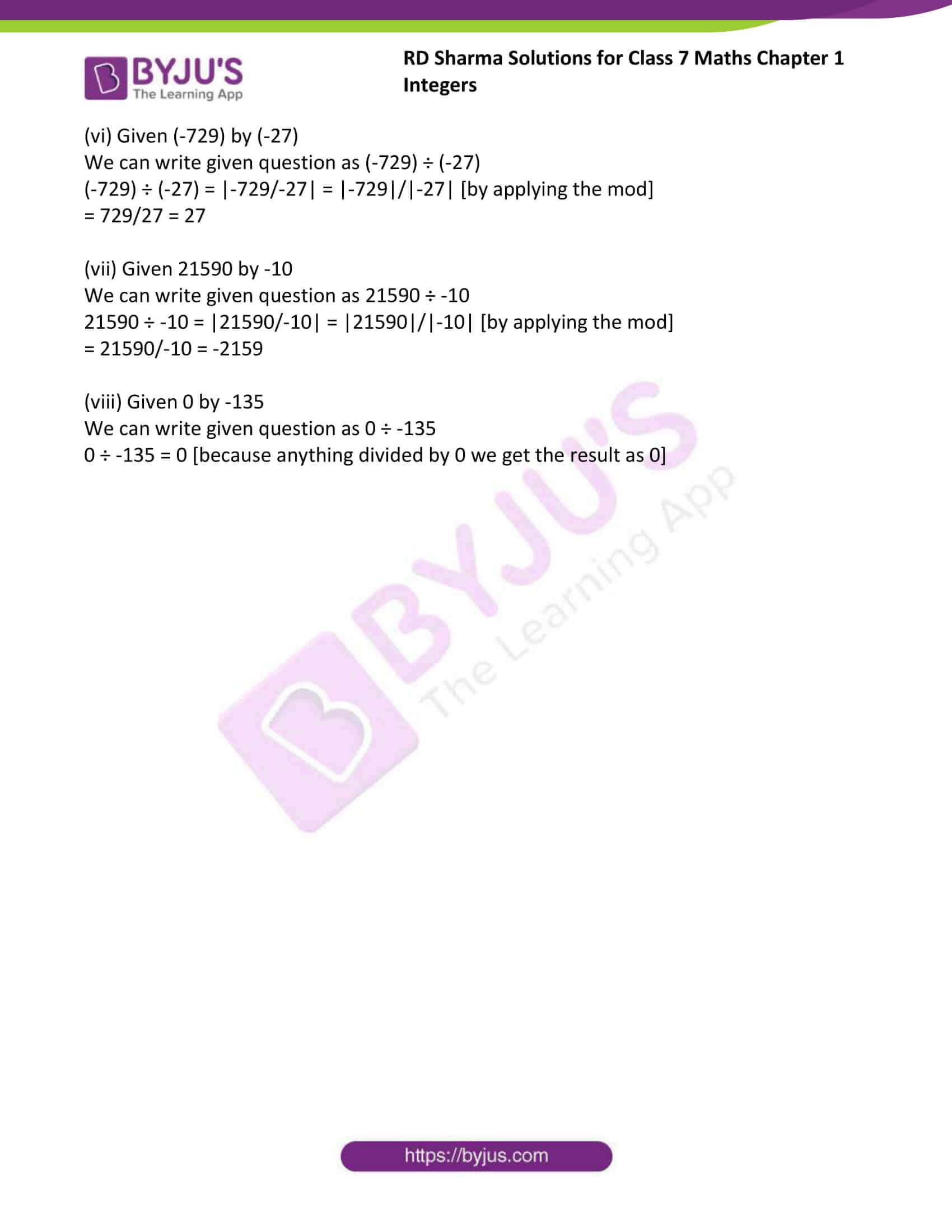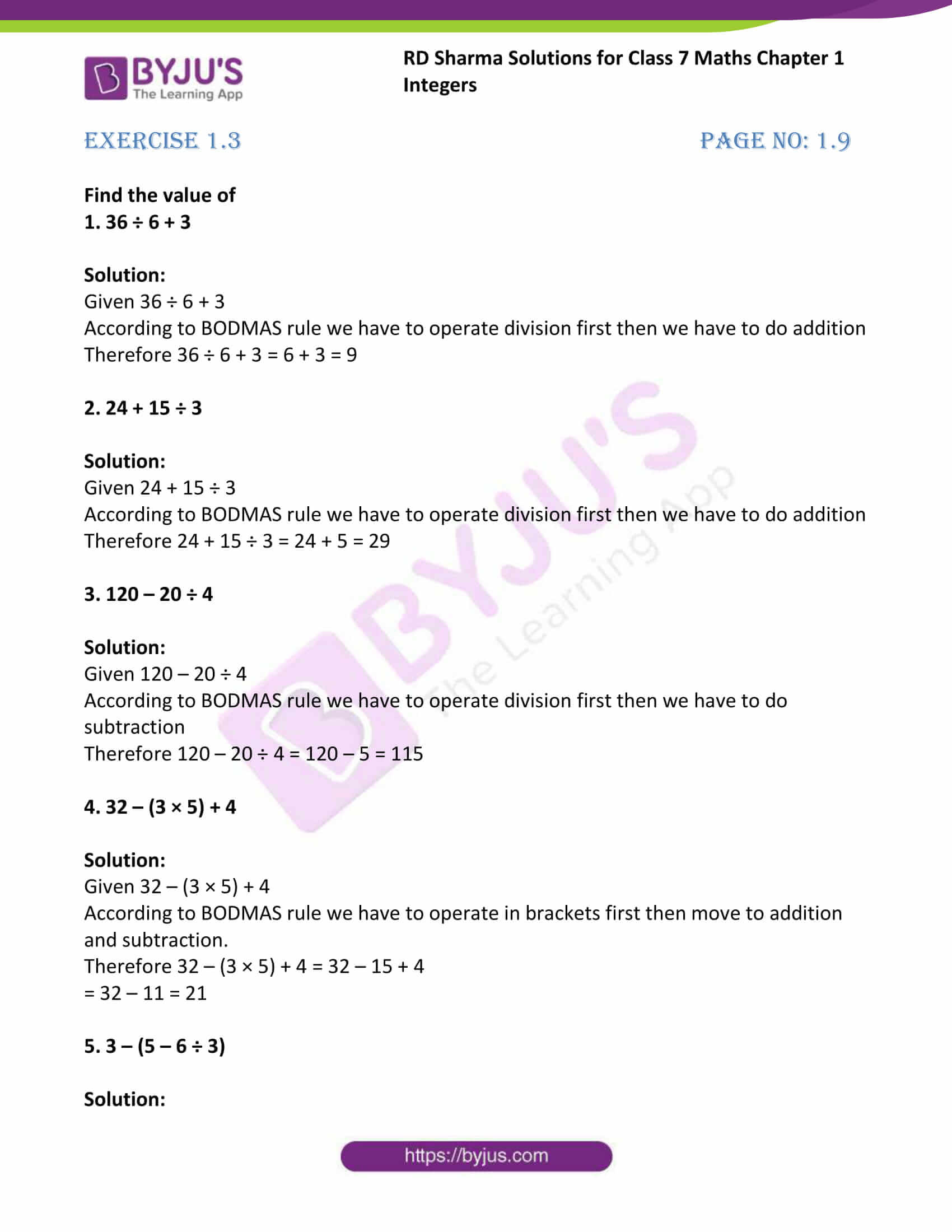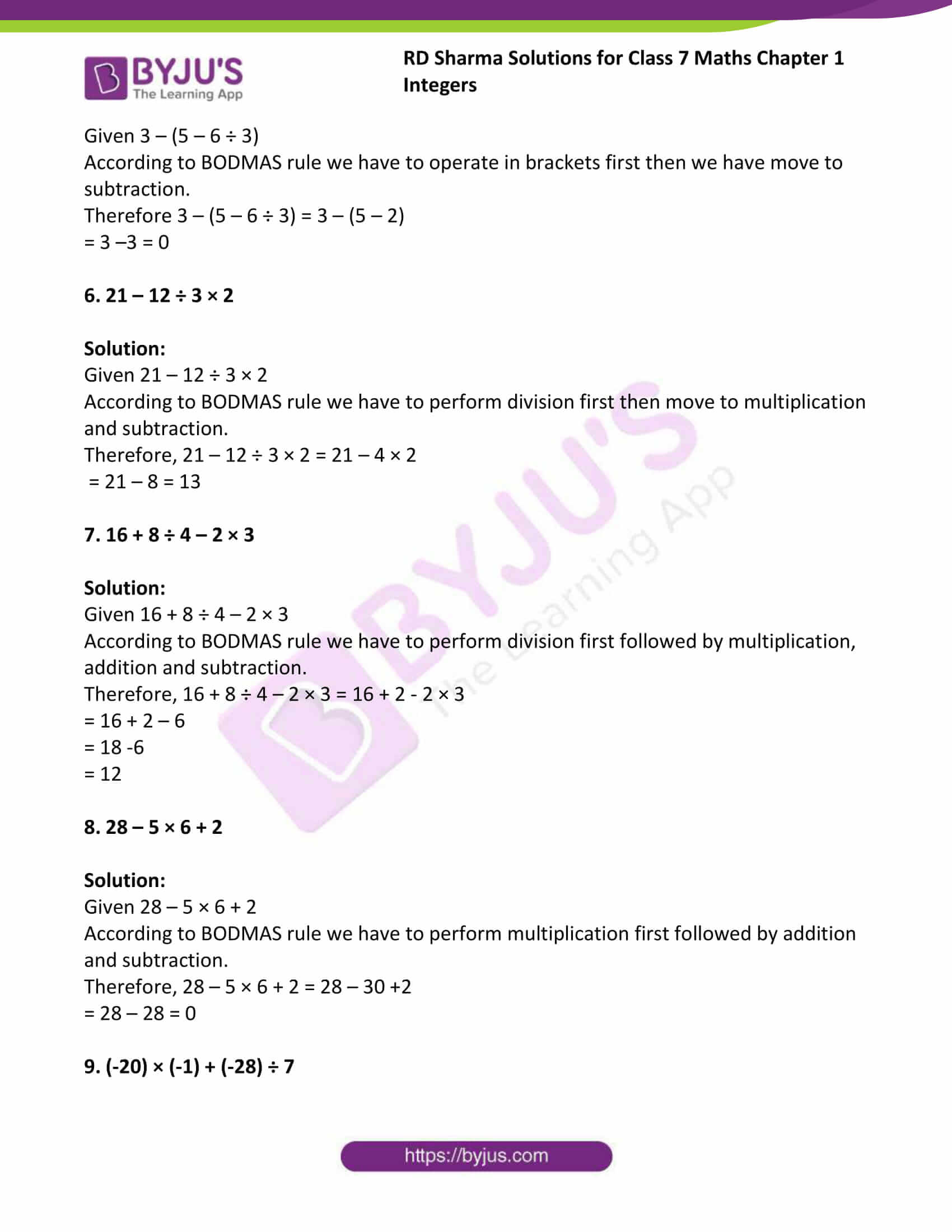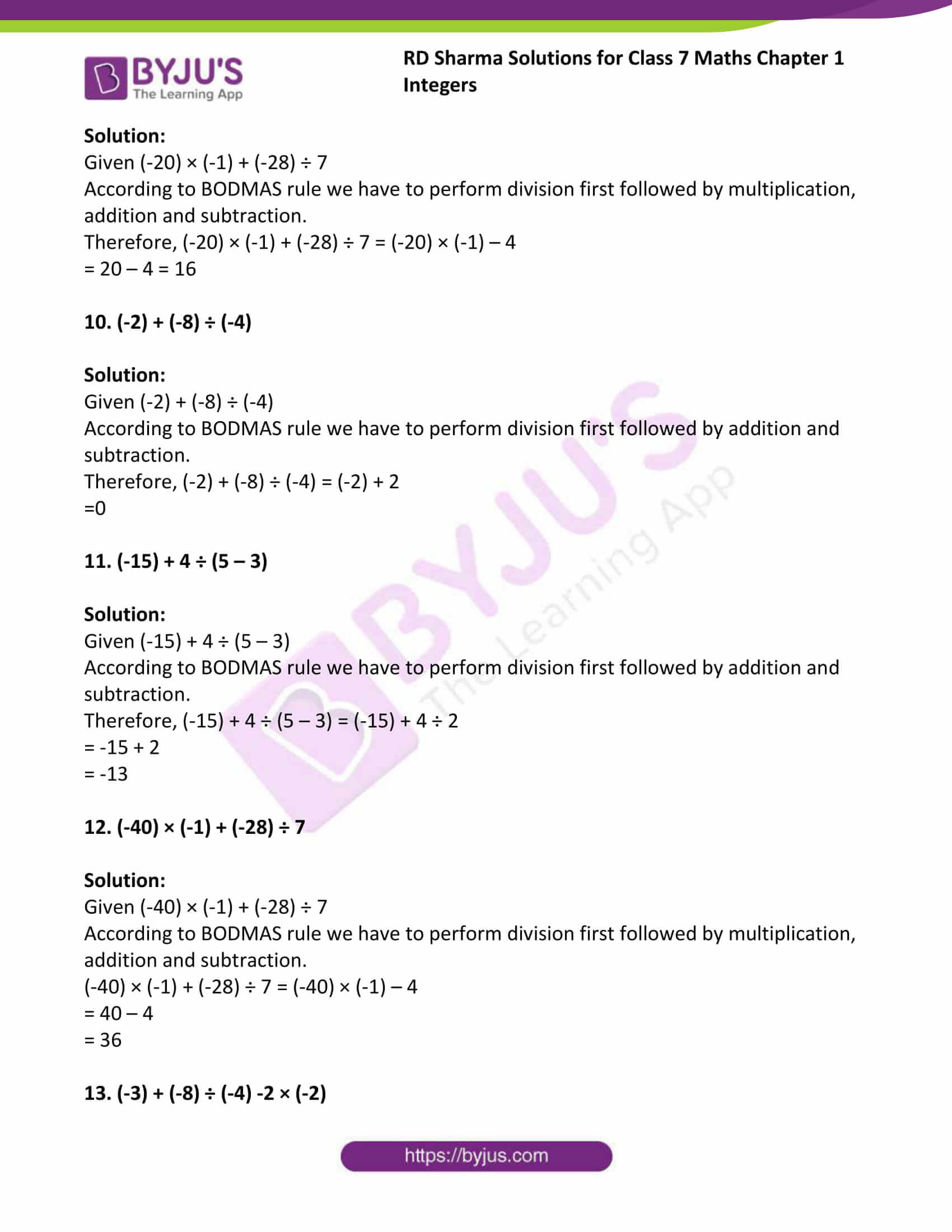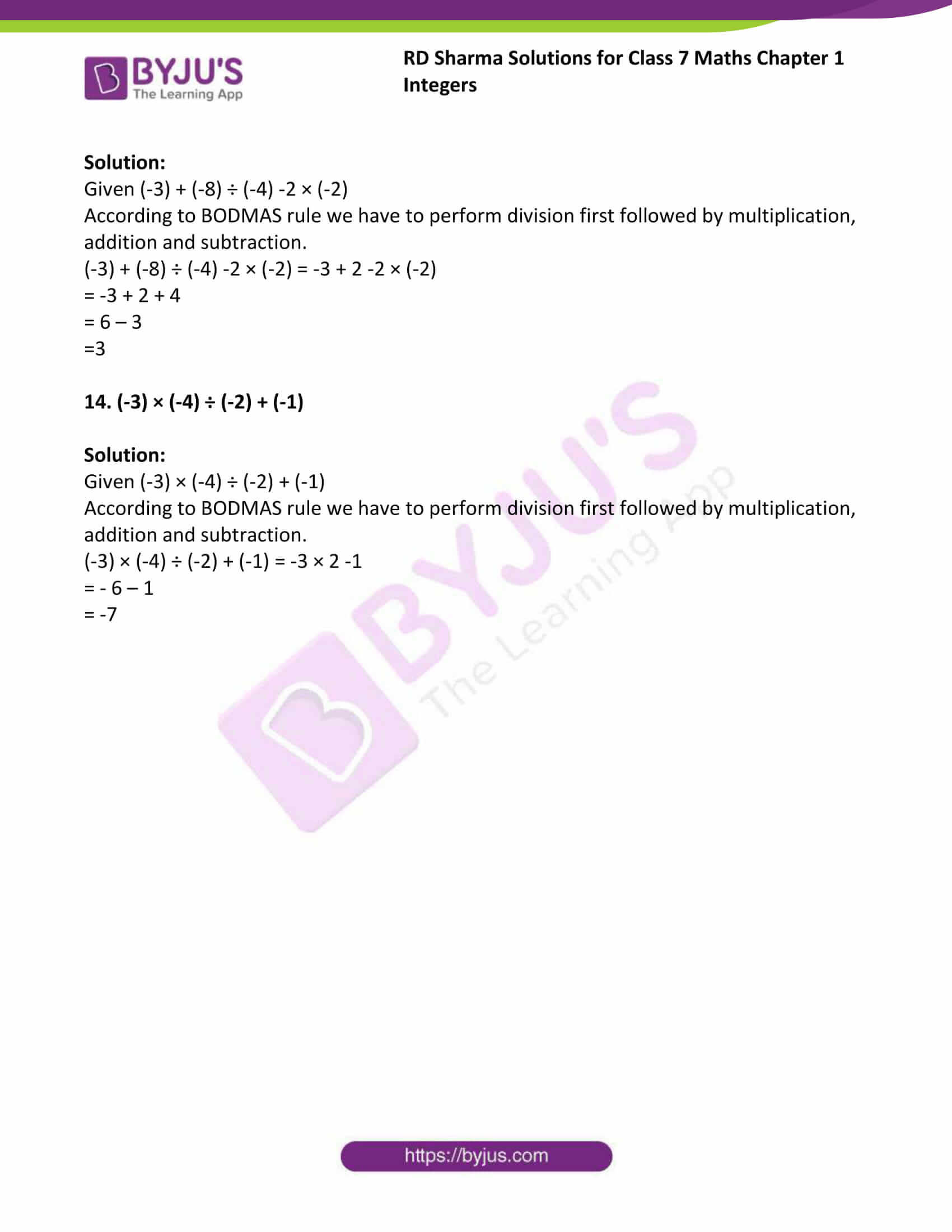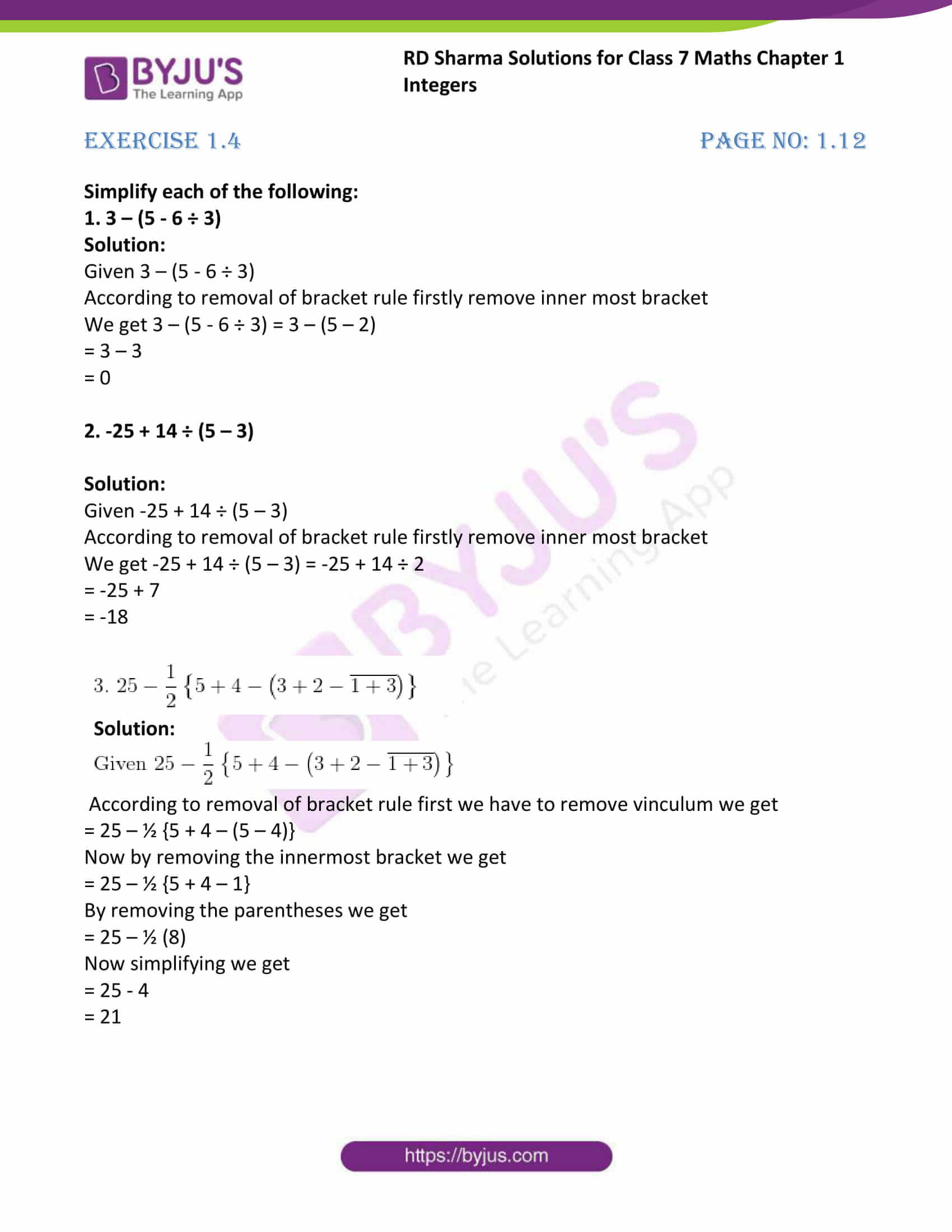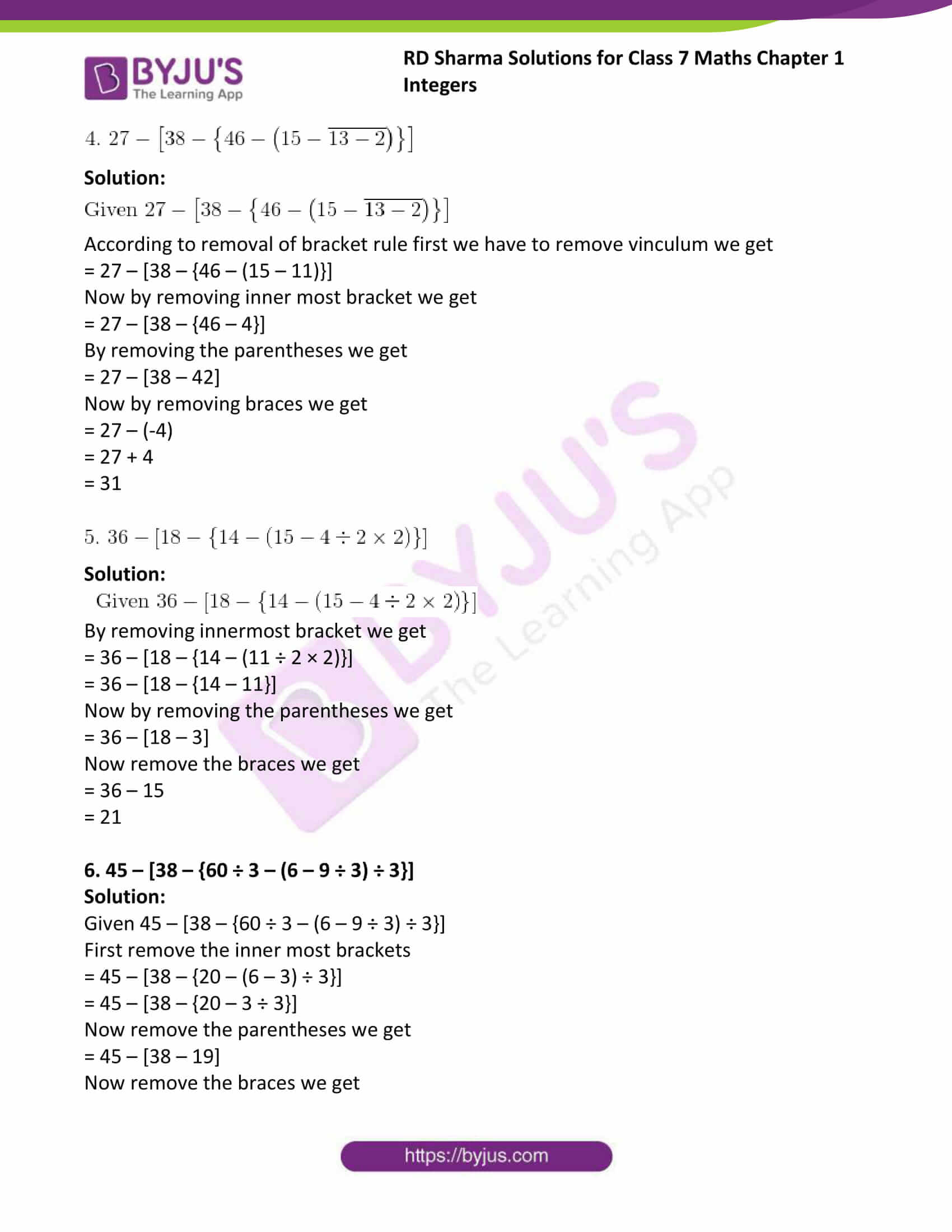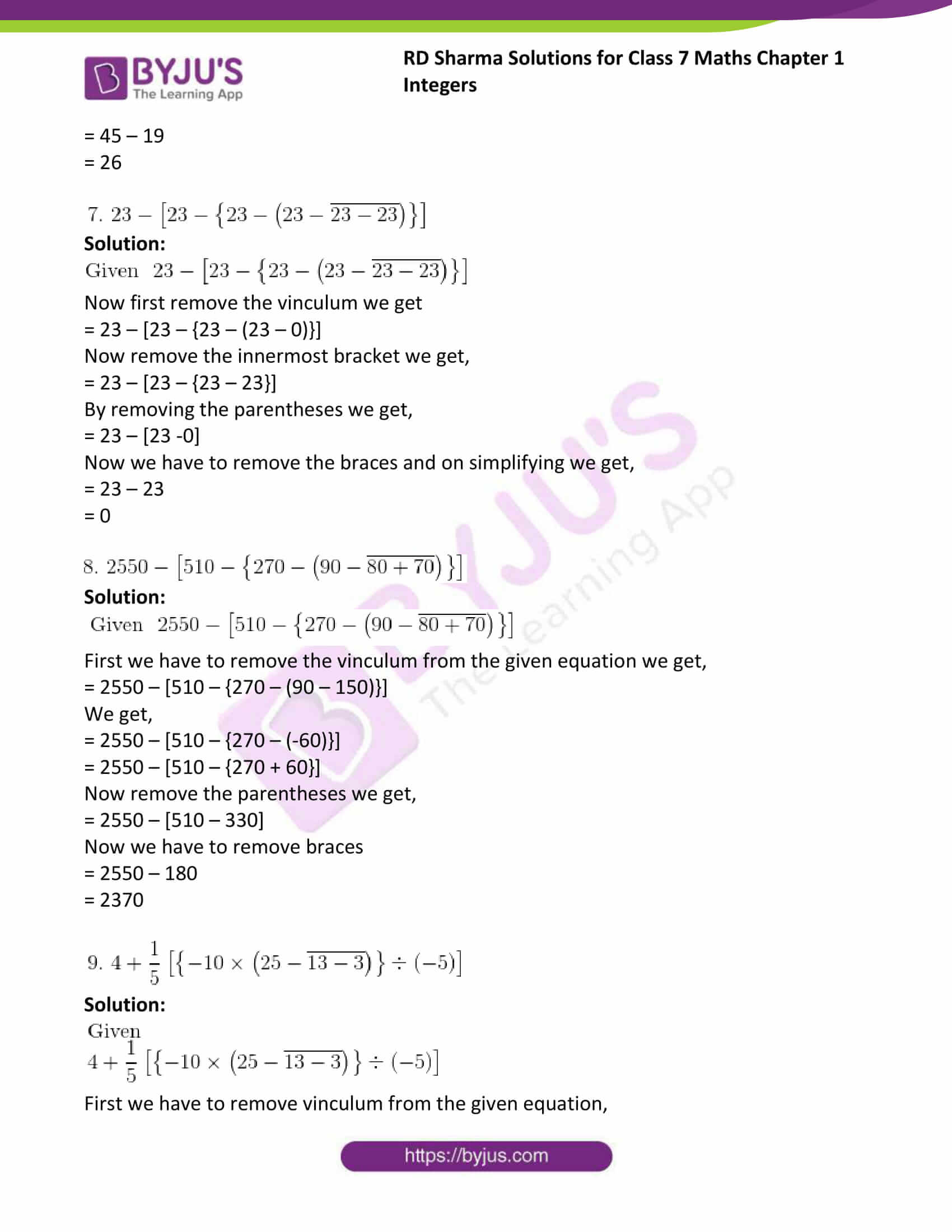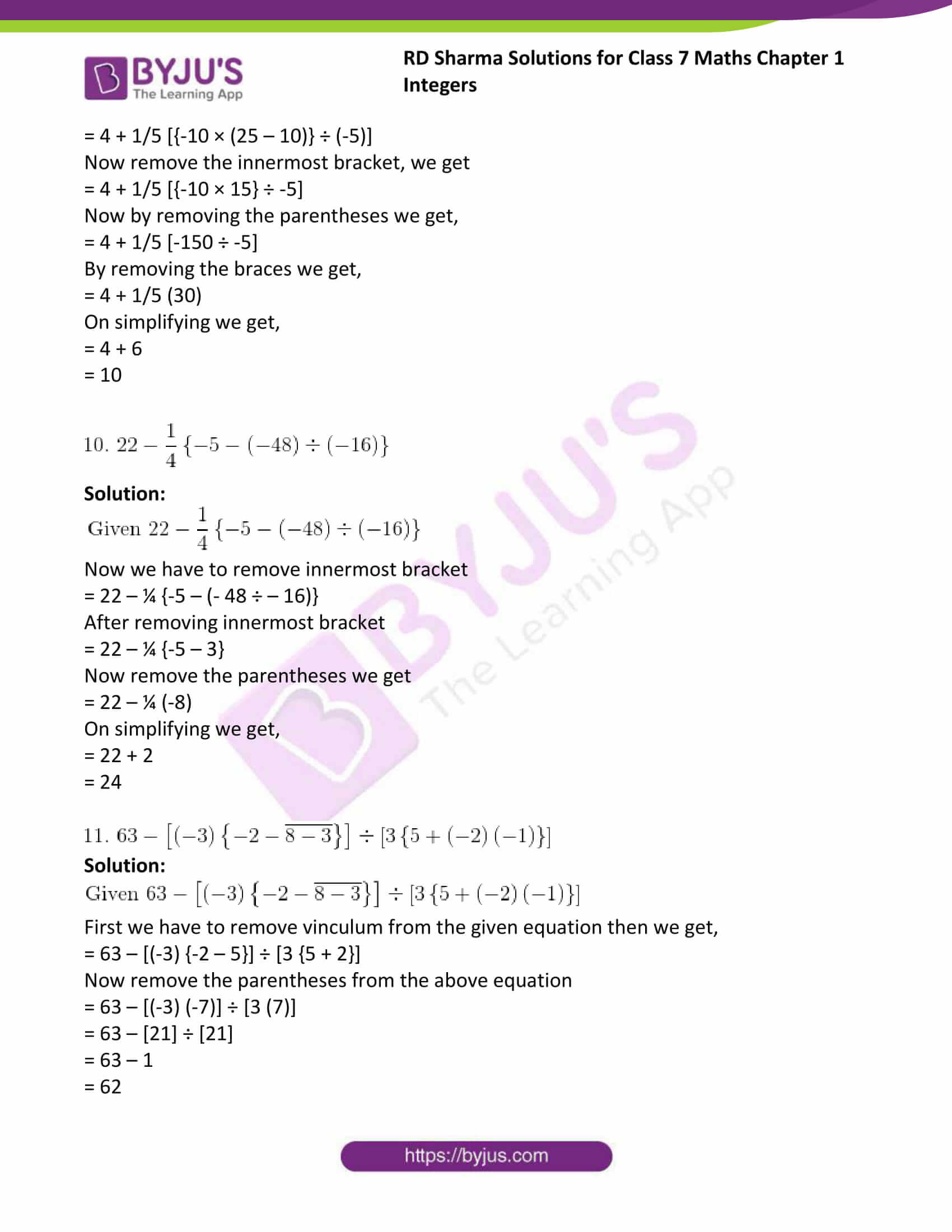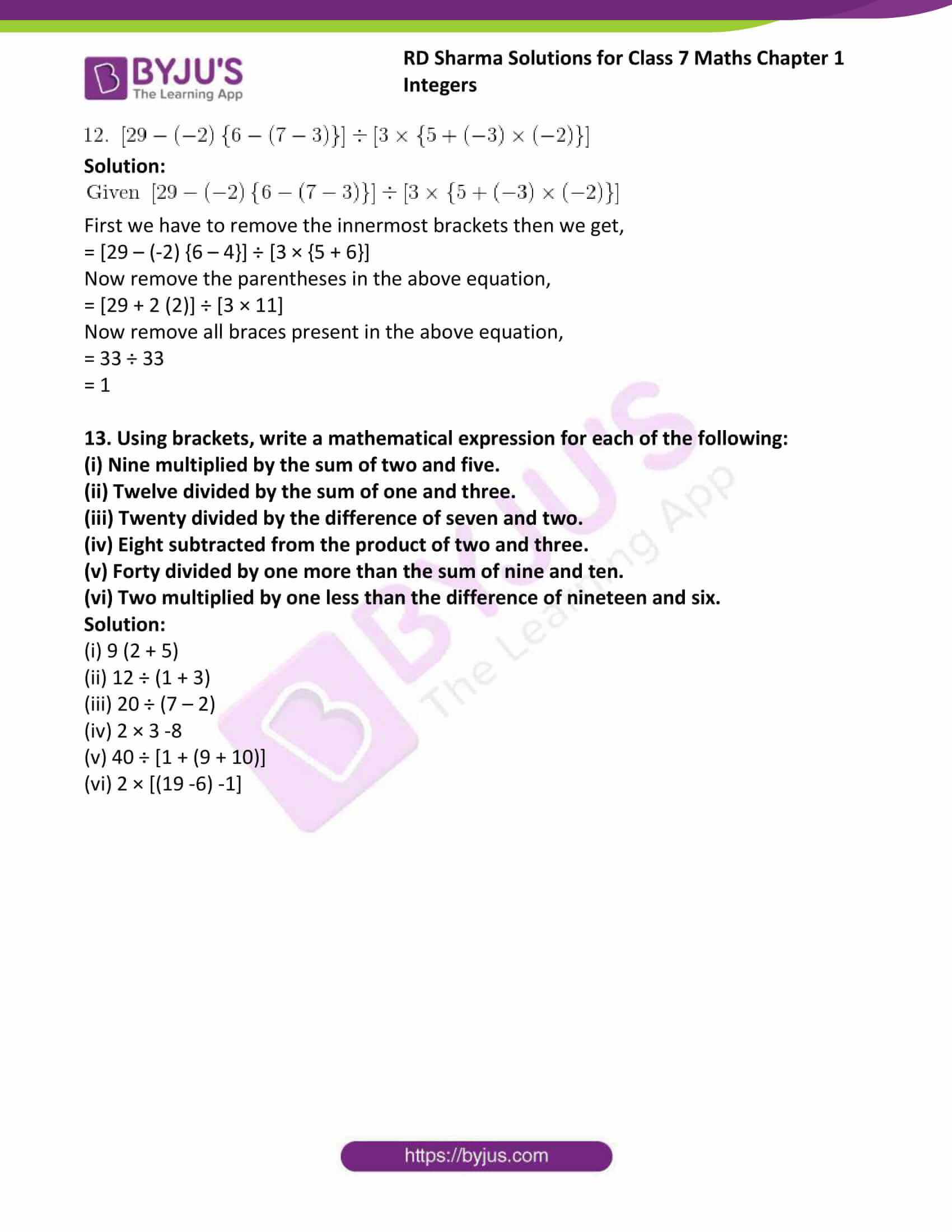### Access answers to Maths RD Sharma Solutions For Class 7 Chapter 1 – Integers

Exercise 1.1 Page No: 1.5

1. Determine each of the following products:

(i) 12 × 7

(ii) (-15) × 8

(iii) (-25) × (-9)

(iv) 125 × (-8)

Solution:

(i) Given 12 × 7

Here we have to find the products of given numbers

12 ×7 = 84

Because the product of two integers of like signs is equal to the product of their absolute values.

(ii) Given (-15) × 8

Here we have to find the products of given numbers

(-15) ×8 = -120

Because the product of two integers of opposite signs is equal to the additive inverse of the product of their absolute values.

(iii) Given (-25) × (-9)

Here we have to find the products of given numbers

(-25) × (-9) = + (25 ×9) = +225

Because the product of two integers of opposite signs is equal to the additive inverse of the product of their absolute values.

(iv) Given 125 × (-8)

Here we have to find the products of given numbers

125 × (-8) = -1000

Because the product of two integers of opposite signs is equal to the additive inverse of the product of their absolute values.

2. Find each of the following products:

(i) 3 × (-8) × 5

(ii) 9 × (-3) × (-6)

(iii) (-2) × 36 × (-5)

(iv) (-2) × (-4) × (-6) × (-8)

Solution:

(i) Given 3 × (-8) ×5

Here we have to find the product of given number.

3 × (-8) × 5 = 3 × (-8 × 5)

=3 × -40 = -120

Since the product of two integers of opposite signs is equal to the additive inverse of the product of their absolute values.

(ii) Given 9 × (-3) × (-6)

Here we have to find the product of given number.

9 × (-3) × (-6) = 9 × (-3 × -6) [∵ the product of two integers of like signs is equal to

the product of their absolute values.]

=9 × +18 = +162

(iii) Given (-2) × 36 × (-5)

Here we have to find the product of given number.

(-2) × 36 × (-5) = (-2 × 36) × -5 [∵ the product of two integers of like signs is equal to

the product of their absolute values.]

=-72 × -5 = +360

(iv) Given (-2) × (-4) × (-6) × (-8)

Here we have to find the product of given number.

(-2) × (-4) × (-6) × (-8) = (-2 × -4) × (-6 × -8) [∵ the product of two integers of like signs is

equal to the product of their absolute values.]

=-8 × -48 = +384

3. Find the value of:

(i) 1487 × 327 + (-487) × 327

(ii) 28945 × 99 – (-28945)

Solution:

(i) Given 1487 × 327 + (-487) × 327

By using the rule of multiplication of integers, we have

1487 × 327 + (-487) × 327 = 486249 – 159249

Since the product of two integers of opposite signs is equal to the additive inverse of the product of their absolute values.

=327000

(ii) Given 28945 × 99 – (-28945)

By using the rule of multiplication of integers, we have

28945 × 99 – (-28945) = 2865555 + 28945

Since the product of two integers of like signs is equal to the product of their absolute values.

=2894500

4. Complete the following multiplication table:

Second number

 First number x -4 -3 -2 -1 0 1 2 3 4 -4 -3 -2 -1 0 1 2 3 4

Is the multiplication table symmetrical about the diagonal joining the upper left corner to the lower right corner?

Solution:

Second number

 First number x -4 -3 -2 -1 0 1 2 3 4 -4 16 12 8 4 0 -4 -8 -12 -16 -3 12 9 6 3 0 -3 -6 -9 -12 -2 8 6 4 2 0 -2 -4 -6 -8 -1 4 3 2 1 0 -1 -2 -3 -4 0 0 0 0 0 0 0 0 0 0 1 -4 -3 -2 -1 0 1 2 3 4 2 -8 -6 -4 -2 0 2 4 6 8 3 -12 -9 -6 -3 0 3 6 9 12 4 -16 -12 -8 -4 0 4 8 12 16

From the table it is clear that, the table is symmetrical about the diagonal joining the upper left corner to the lower right corner.

5. Determine the integer whose product with ‘-1’ is

(i) 58

(ii) 0

(iii) -225

Solution:

(i) Given 58

Here we have to find the integer which is multiplied by -1

We get, 58 × -1 = -58

Since the product of two integers of opposite signs is equal to the additive inverse of the product of their absolute values.

(ii) Given 0

Here we have to find the integer which is multiplied by -1

We get, 0 × -1 = 0 [because anything multiplied with 0 we get 0 as their result]

(iii) Given -225

Here we have to find the integer which is multiplied by -1

We get, -225 × -1 = 225

Since the product of two integers of like signs is equal to the product of their absolute values.

Exercise 1.2 Page No: 1.8

1. Divide:

(i) 102 by 17

(ii) -85 by 5

(iii) -161 by -23

(iv) 76 by -19

(v) 17654 by -17654

(vi) (-729) by (-27)

(vii) 21590 by -10

(viii) 0 by -135

Solution:

(i) Given 102 by 17

We can write given question as 102 ÷ 17

102 ÷ 17 = |102/17| = |102|/|17| [by applying the mod]

= 102/17 = 6

(ii) Given -85 by 5

We can write given question as -85 ÷ 5

-85 ÷ 5 = |-85/5| = |-85|/|5| [by applying the mod]

= -85/5 = -17

(iii) Given -161 by -23

We can write given question as -161 ÷ -23

-161 ÷ -23 = |-161/-23| = |-161|/|-23| [by applying the mod]

= 161/23 = 7

(iv) Given 76 by -19

We can write given question as 76 ÷ -19

76 ÷ -19 = |76/-19| = |76|/|-19| [by applying the mod]

= 76/-19 = -4

(v) Given 17654 by -17654

We can write given question as 17654 ÷ -17654

17654 ÷ -17654 = |17654/-17654| = |17654|/|-17654| [by applying the mod]

= 17654/-17654 = -1

(vi) Given (-729) by (-27)

We can write given question as (-729) ÷ (-27)

(-729) ÷ (-27) = |-729/-27| = |-729|/|-27| [by applying the mod]

= 729/27 = 27

(vii) Given 21590 by -10

We can write given question as 21590 ÷ -10

21590 ÷ -10 = |21590/-10| = |21590|/|-10| [by applying the mod]

= 21590/-10 = -2159

(viii) Given 0 by -135

We can write given question as 0 ÷ -135

0 ÷ -135 = 0 [because anything divided by 0 we get the result as 0]

Exercise 1.3 Page No: 1.9

Find the value of

1. 36 ÷ 6 + 3

Solution:

Given 36 ÷ 6 + 3

According to BODMAS rule we have to operate division first then we have to do addition

Therefore 36 ÷ 6 + 3 = 6 + 3 = 9

2. 24 + 15 ÷ 3

Solution:

Given 24 + 15 ÷ 3

According to BODMAS rule we have to operate division first then we have to do addition

Therefore 24 + 15 ÷ 3 = 24 + 5 = 29

3. 120 – 20 ÷ 4

Solution:

Given 120 – 20 ÷ 4

According to BODMAS rule we have to operate division first then we have to do subtraction

Therefore 120 – 20 ÷ 4 = 120 – 5 = 115

4. 32 – (3 × 5) + 4

Solution:

Given 32 – (3 × 5) + 4

According to BODMAS rule we have to operate in brackets first then move to addition and subtraction.

Therefore 32 – (3 × 5) + 4 = 32 – 15 + 4

= 32 – 11 = 21

5. 3 – (5 – 6 ÷ 3)

Solution:

Given 3 – (5 – 6 ÷ 3)

According to BODMAS rule we have to operate in brackets first then we have move to subtraction.

Therefore 3 – (5 – 6 ÷ 3) = 3 – (5 – 2)

= 3 –3 = 0

6. 21 – 12 ÷ 3 × 2

Solution:

Given 21 – 12 ÷ 3 × 2

According to BODMAS rule we have to perform division first then move to multiplication and subtraction.

Therefore, 21 – 12 ÷ 3 × 2 = 21 – 4 × 2

= 21 – 8 = 13

7. 16 + 8 ÷ 4 – 2 × 3

Solution:

Given 16 + 8 ÷ 4 – 2 × 3

According to BODMAS rule we have to perform division first followed by multiplication, addition and subtraction.

Therefore, 16 + 8 ÷ 4 – 2 × 3 = 16 + 2 – 2 × 3

= 16 + 2 – 6

= 18 -6

= 12

8. 28 – 5 × 6 + 2

Solution:

Given 28 – 5 × 6 + 2

According to BODMAS rule we have to perform multiplication first followed by addition and subtraction.

Therefore, 28 – 5 × 6 + 2 = 28 – 30 +2

= 28 – 28 = 0

9. (-20) × (-1) + (-28) ÷ 7

Solution:

Given (-20) × (-1) + (-28) ÷ 7

According to BODMAS rule we have to perform division first followed by multiplication, addition and subtraction.

Therefore, (-20) × (-1) + (-28) ÷ 7 = (-20) × (-1) – 4

= 20 – 4 = 16

10. (-2) + (-8) ÷ (-4)

Solution:

Given (-2) + (-8) ÷ (-4)

According to BODMAS rule we have to perform division first followed by addition and subtraction.

Therefore, (-2) + (-8) ÷ (-4) = (-2) + 2

=0

11. (-15) + 4 ÷ (5 – 3)

Solution:

Given (-15) + 4 ÷ (5 – 3)

According to BODMAS rule we have to perform division first followed by addition and subtraction.

Therefore, (-15) + 4 ÷ (5 – 3) = (-15) + 4 ÷ 2

= -15 + 2

= -13

12. (-40) × (-1) + (-28) ÷ 7

Solution:

Given (-40) × (-1) + (-28) ÷ 7

According to BODMAS rule we have to perform division first followed by multiplication, addition and subtraction.

(-40) × (-1) + (-28) ÷ 7 = (-40) × (-1) – 4

= 40 – 4

= 36

13. (-3) + (-8) ÷ (-4) -2 × (-2)

Solution:

Given (-3) + (-8) ÷ (-4) -2 × (-2)

According to BODMAS rule we have to perform division first followed by multiplication, addition and subtraction.

(-3) + (-8) ÷ (-4) -2 × (-2) = -3 + 2 -2 × (-2)

= -3 + 2 + 4

= 6 – 3

=3

14. (-3) × (-4) ÷ (-2) + (-1)

Solution:

Given (-3) × (-4) ÷ (-2) + (-1)

According to BODMAS rule we have to perform division first followed by multiplication, addition and subtraction.

(-3) × (-4) ÷ (-2) + (-1) = -3 × 2 -1

= – 6 – 1

= -7

Exercise 1.4 Page No: 1.12

Simplify each of the following:

1. 3 – (5 – 6 ÷ 3)

Solution:

Given 3 – (5 – 6 ÷ 3)

According to removal of bracket rule firstly remove inner most bracket

We get 3 – (5 – 6 ÷ 3) = 3 – (5 – 2)

= 3 – 3

= 0

2. -25 + 14 ÷ (5 – 3)

Solution:

Given -25 + 14 ÷ (5 – 3)

According to removal of bracket rule firstly remove inner most bracket

We get -25 + 14 ÷ (5 – 3) = -25 + 14 ÷ 2

= -25 + 7

= -18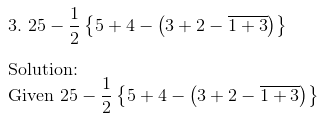Solution:According to removal of bracket rule first we have to remove vinculum we get

= 25 – ½ {5 + 4 – (5 – 4)}

Now by removing the innermost bracket we get

= 25 – ½ {5 + 4 – 1}

By removing the parentheses we get

= 25 – ½ (8)

Now simplifying we get

= 25 – 4

= 21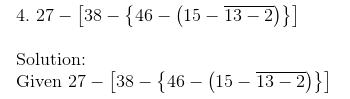Solution:According to removal of bracket rule first we have to remove vinculum we get

= 27 – [38 – {46 – (15 – 11)}]

Now by removing inner most bracket we get

= 27 – [38 – {46 – 4}]

By removing the parentheses we get

= 27 – [38 – 42]

Now by removing braces we get

= 27 – (-4)

= 27 + 4

= 31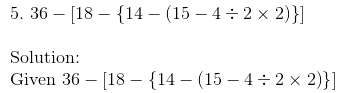Solution:By removing innermost bracket we get

= 36 – [18 – {14 – (11 ÷ 2 × 2)}]

= 36 – [18 – {14 – 11}]

Now by removing the parentheses we get

= 36 – [18 – 3]

Now remove the braces we get

= 36 – 15

= 21

6. 45 – [38 – {60 ÷ 3 – (6 – 9 ÷ 3) ÷ 3}]

Solution:

Given 45 – [38 – {60 ÷ 3 – (6 – 9 ÷ 3) ÷ 3}]

First remove the inner most brackets

= 45 – [38 – {20 – (6 – 3) ÷ 3}]

= 45 – [38 – {20 – 3 ÷ 3}]

Now remove the parentheses we get

= 45 – [38 – 19]

Now remove the braces we get

= 45 – 19

= 26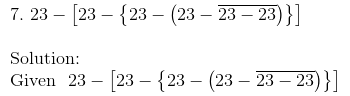Solution:Now first remove the vinculum we get

= 23 – [23 – {23 – (23 – 0)}]

Now remove the innermost bracket we get,

= 23 – [23 – {23 – 23}]

By removing the parentheses we get,

= 23 – [23 -0]

Now we have to remove the braces and on simplifying we get,

= 23 – 23

= 0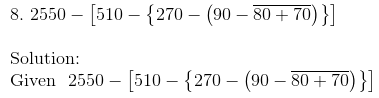Solution:First we have to remove the vinculum from the given equation we get,

= 2550 – [510 – {270 – (90 – 150)}]

We get,

= 2550 – [510 – {270 – (-60)}]

= 2550 – [510 – {270 + 60}]

Now remove the parentheses we get,

= 2550 – [510 – 330]

Now we have to remove braces

= 2550 – 180

= 2370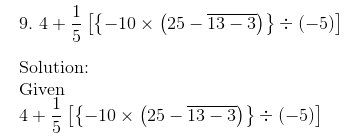Solution:First we have to remove vinculum from the given equation,

= 4 + 1/5 [{-10 × (25 – 10)} ÷ (-5)]

Now remove the innermost bracket, we get

= 4 + 1/5 [{-10 × 15} ÷ -5]

Now by removing the parentheses we get,

= 4 + 1/5 [-150 ÷ -5]

By removing the braces we get,

= 4 + 1/5 (30)

On simplifying we get,

= 4 + 6

= 10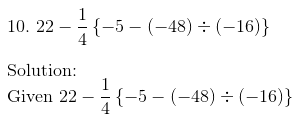Solution:Now we have to remove innermost bracket

= 22 – ¼ {-5 – (- 48 ÷ – 16)}

After removing innermost bracket

= 22 – ¼ {-5 – 3}

Now remove the parentheses we get

= 22 – ¼ (-8)

On simplifying we get,

= 22 + 2

= 24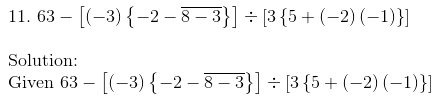Solution:First we have to remove vinculum from the given equation then we get,

= 63 – [(-3) {-2 – 5}] ÷ [3 {5 + 2}]

Now remove the parentheses from the above equation

= 63 – [(-3) (-7)] ÷ [3 (7)]

= 63 –  ÷ 

= 63 – 1

= 62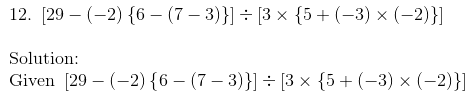Solution:First we have to remove the innermost brackets then we get,

= [29 – (-2) {6 – 4}] ÷ [3 × {5 + 6}]

Now remove the parentheses in the above equation,

= [29 + 2 (2)] ÷ [3 × 11]

Now remove all braces present in the above equation,

= 33 ÷ 33

= 1

13. Using brackets, write a mathematical expression for each of the following:

(i) Nine multiplied by the sum of two and five.

(ii) Twelve divided by the sum of one and three.

(iii) Twenty divided by the difference of seven and two.

(iv) Eight subtracted from the product of two and three.

(v) Forty divided by one more than the sum of nine and ten.

(vi) Two multiplied by one less than the difference of nineteen and six.

Solution:

(i) 9 (2 + 5)

(ii) 12 ÷ (1 + 3)

(iii) 20 ÷ (7 – 2)

(iv) 2 × 3 -8

(v) 40 ÷ [1 + (9 + 10)]

(vi) 2 × [(19 -6) -1]

## RD Sharma Solutions For Class 7 Maths Chapter 1 – Integers

Chapter 1, Integers contains four exercises. RD Sharma Solutions are given here which include the answers to all the questions present in these exercises. Let us have a look at some of the concepts that are being discussed in this Chapter.

• Multiplication of integers
• Properties of multiplication
• Division of integers
• Properties of division
• Operator precedence
• Use of brackets
• Removal of brackets

### Chapter brief of RD Sharma Solutions for Class 7 Maths Chapter 1 – Integers

Chapter 1 – Integers primarily covers three topics, which are multiplication and division of integers and operator precedence. It also explains how to use and remove the brackets. Integers can be defined as a ‘’number that can be written without a fractional component”. Here, the students will be thorough about the precedence of fundamental operations of addition, subtraction, multiplication and division.